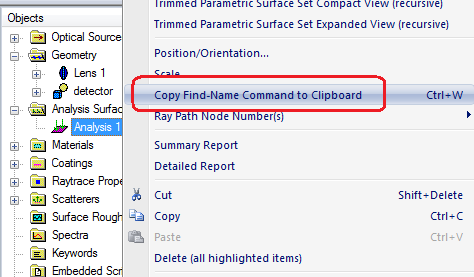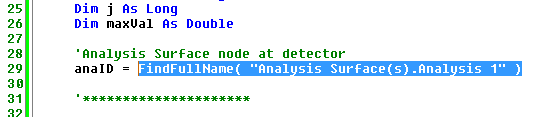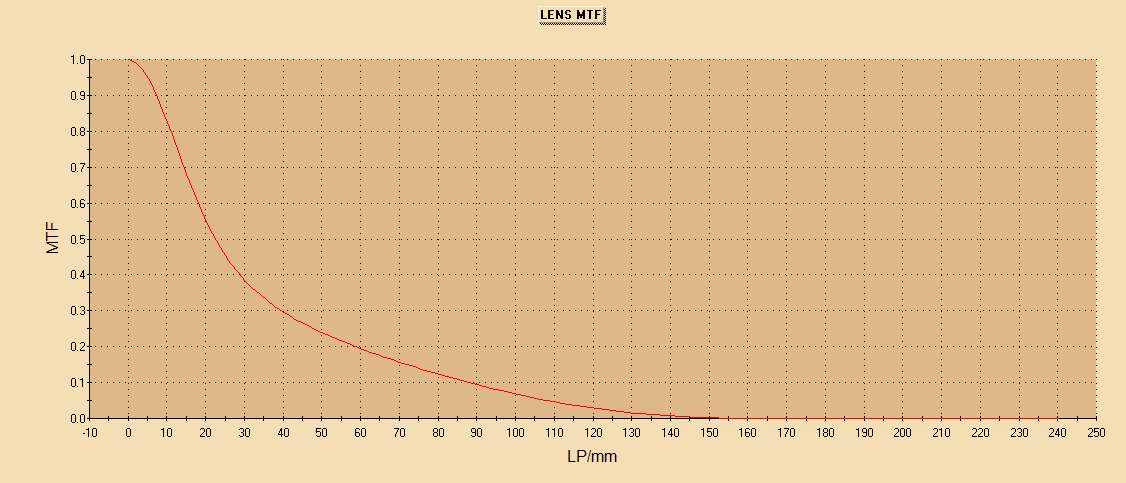# Calculating MTF

The Modulation Transfer function (MTF) is commonly used to describe the performance of an imaging system, specifically how the contrast varies with spatial frequency. It is defined as the normalized Fourier Transform of the Point Spread Function. This standalone FRED script can be run after a raytrace to automatically calculate MTF and display it in a 2D plot. An example FRED document is also provided with this article.

The script is a standalone script that is intended for use with any FRED model for which the user needs to calculate the MTF. However, it should be noted that this script assumes the raytrace has already been performed and that the source is coherent.

To customize the script to work with a specific model, the user needs to specify the Analysis Surface located at the image plane. This is easily done by right-clicking on the Analysis Surface in the Document Tree and selecting Copy Find-Name Command to Clipboard, then pasting into line 29 of the script.The MTF is the normalized Fourier Transform of the Point Spread Function. Therefore the Main subroutine of the script begins by calculating the Irradiance Spread Function at the user-specified Analysis Surface using the the IrradianceToARN function.

Then, in preparation for the FFT, the subroutine uses the ARNCreate2DComplexFromDouble function to convert the numerical Irradiance data to complex values with null imaginary components. This is a prerequisite for the following ARNFFT2D function, which performs the Fourier Transform and creates a new ARN of the transformed data.

```    'Calculate the Irradiance at the detector...

'...convert the single value data to complex pairs with null imaginary values...
arnNode = ARNCreate2DComplexFromDouble( arnNode )

'...perform the Fourier Transform
ARNFFT2D arnNode
<br>
```

To display a 2D plot of the MTF, the positive x data along the y = Y/2 center line is obtained by iterating through the appropriate data points of the ARN and retrieving both the X-axis value (ARNGetAAxisValueAt) and the amplitude of the complex values obtained from the ARNGetCellValueAsDoubleArray2D subroutine.

```'Set the size of the arrays based on the number of data points
numVals = ARNGetAAxisDim( arnNode ) / 2
ReDim xdata( numVals - 1)
ReDim ydata( numVals - 1)

'Loop over the values on the y = Y/2 center line and grab the data
maxVal = 0
For j = 0 To numVals - 1
xdata(j) = ARNGetAAxisValueAt( arnNode, j + numVals )

ARNGetCellValueAsDoubleArray2D arnNode, j + numVals, numVals, celldata()
ydata(j) = ( celldata(0)^2 + celldata(1)^2 )^0.5

'make note of the maximum value for the normalization (lines 66-69 below)
If ydata(j) > maxVal Then
maxVal = ydata(j)
End If
Next j<br>
```

Before plotting the 2D plot of the MTF, the data is normalized to a maximum value of unity using the maxVal variable populated during the above loop.

In this script, a separate subroutine, Plot2D, has been created outside of the Main subroutine. The x and y data arrays are passed to it, along with strings defining the plot title and axes labels and a save flag.

```    'Plot the data in 2D Chart using the subroutine defined below
Plot2D(xdata(), ydata(), "LENS MTF", "LP/mm", "MTF", True)  'title, xlabel, ylabel, save Image<br>
```

Immediately in the Plot2D subroutine, an instance of the 2D Plot is created, and then the data series is added. This is done with the AddUser2DPlot and AddUser2DPlotSeries functions. The remainder of the functions and subroutines such as SetUser2DPlotGraphTitle should be self-explanatory, but details are provided in FRED's Online Help.Associated FRED script:  CalculatingMTF.frs

Associated FRED file:  lensMTF.frd